# HC Verma Solutions Class 11 Chapter 14 Some Mechanical Properties of Matter

HC Verma Class 11 Solutions Chapter 14 Some Mechanical Properties of Matter is a very important chapter and consists of questions which are frequent in competitive examinations. While studying this chapter students will come across questions related to finding stress, strain and compression etc. They will have to solve questions related to comparing compression, strain, and stress of metals such as steel and copper. In order to help, students master the concepts discussed in chapter 14 of HC Verma book vol 1 and develop better problems skills we are offering free solutions on this page along with the pdf to study offline. These solutions will also help students boost their preparation for JEE Main and Advanced. With these study material, students will further get to learn solving problems such as:

• Finding the tension in the string when the load is suspended.
• Questions on Lateral displacement, pressure and surface energy, etc.
• Solve problems on the viscous force, hydrostatic force, the terminal velocity of raindrops and more.

The HC Verma solutions contain answers that are arranged in a systematic manner for easy navigation and learning.

Some of the important topics that students will also learn in this chapter are;

1. Molecular Structure of a Material
2. Elasticity
3. Stress
4. Strain
5. Hooke’s Law and the Modulii of Elasticity
6. The relation between Longitudinal Stress and Strain
7. Elastic Potential Energy of a Strained Body
8. Determination of Young’s Modulus in Laboratory
9. Surface Tension
10. Surface Energy
11. Excess Pressure Inside a Drop
12. Excess Pressure/ in a Soap Bubble
13. Contact Angle
14. Rise of Liquid in a Capillary Tube
15. Viscosity
16. Flow through a Narrow Tube: Poiseuille’s Equation
17. Stokes’ Law
18. Terminal Velocity
19. Measuring the Coefficient of Viscosity by Stokes’ Method
20. Critical Velocity and Reynolds Number

## Class 11 Important Questions In Chapter 14

1. What will happen to a spring’s elastic potential energy when it is dissolved in acid in a compressed state?

2. Let’s take a glass capillary tube and dip it at one end in water. We will notice the water rise in the tube. This also results in increased gravitational potential energy. What can you state about this action considering the conservation of energy? Does it violate it?

3. What happens when small drops of water are used to form a bigger drop?

(a) energy is absorbed (b) energy is liberated (c) energy is either liberated or absorbed as per the nature of the liquid (d) energy is neither liberated or absorbed

4. A falling solid sphere has a terminal velocity of 20 m/s in air. What will happen if it falls in a vacuum?

(a) terminal velocity will be less than 20 m/s (b) terminal velocity will be more than 20 m/s (c) terminal velocity will be 20 m/s (d) Terminal velocity does not exist.

5. To which state does the property of viscosity belong to;

(a) Solids (b) Liquids (c) Both (d) Liquids and Gases

## HC Verma Solutions Vol 1 Some Mechanical Properties of Matter Chapter 14

Question 1: A load of 10 kg is suspended by a metal wire 3 m long and having a cross-sectional area 4 mm2. Find (a) the stress (b) the strain and (c) the elongation. Young modulus of the metal is 2.0 × 1011 N m-2.

Solution:

(a) Stress = Force/Area = mg/A

= (10×10)/(4×10-6)

= 2.5 x 10-7 N/m2

(b) Strain = Stress/Y

= [2.5 x 10-7]/[2×1011]

= 1.25 x 10-4 m

(c) Let elongation be I

I = L x strain = 3.75 x 10-4 m

Question 2: A vertical metal cylinder of radius 2 cm and length 2 m is fixed at the lower end and a load of 100 kg is put on it. Find (a) the stress (b) the strain and (c) the compression of the cylinder. Young modulus of the metal = 2 x 1011 N m-2.

Solution:

Area of cross-section of the cylinder = A = πr2

= π x 0.022

= 4π x 10-4 m2

(a) stress = Force/Area

= [10×10]/[4π x 10-4]

= 7.96 x 105 N/m2

(b) Strain = Stress/Y

= [7.96 x 105]/[2×1011]

= 3.98 x 10-6

(c) Let compression be I

I = 2 x (3.98 x 10-6) = 8 x 10-6 m (approx)

Question 3: The elastic limit of steel is 8 × 108 N m-2 and its Young modulus 2x 1011 Nm-2. Find the maximum elongation of a half-meter steel wire that can be given without exceeding the elastic limit.

Solution:

The elastic limit of steel is 8 × 108 N m-2 and its Young modulus 2 × 1011 N m-2.

Strain = Stress/Y and Stress = I/L

=> Stress/Y = I/L

=> I = (stress L)/Y

= [8×108 x0.5]/[2×1011]

= 2 x 10-3 m

Question 4: A steel wire and a copper wire of equal length and equal cross-sectional area are joined end to end and the combination is subjected to a tension. Find the ratio of (a) the stresses developed in the two wires and (b) the strains developed. Y of steel = 2 × 1011 N m-2. Y of copper = 1.3 × 1011 N m-2.

Solution:

(a) Let the stress in steel wire be σs and in copper σc

Now, σs = F/A and σc = F/A

=> σsc = 1

(b) Strain, ε = σ/Y

Strain in the steel wire = εs = [F/A]/[2×1011]

and strain in the copper wire = εc = [F/A]/[1.3×1011]

Hence, εcs = 20/13

Question 5: In figure (below) the upper wire is made of steel and the lower of copper. The wires have equal cross section. Find the ratio of the longitudinal strains developed in the two wires.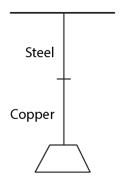Solution:

[Strain in the steel wire]/[strain in the copper wire] = [2×1011]/[1.3×1011]

= 20/13

= 1.54 (approx)

Question 6: The two wires shown in figure (below) are made of the same material which has a breaking stress of 8 × 108 N m-2. The area of cross section of the upper wire is 0.006 cm2 and that of the lower wire is 0.003 cm2. The mass m1 = 10 kg, m2 =20 kg and the hanger is light. (a) Find the maximum load that can be put on the hanger without breaking a wire. Which wire will break first if the load is increased? (b) Repeat the above part if m1 = 10 kg and m2 = 36 kg.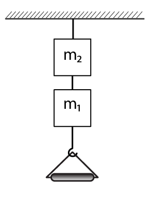Solution:

(a) Stress on the lower wire = σ = [(total weight of lower wire)g]/[Area of cross section of upper wire]

= [m+m1]g/A1

Let say, A1 = Area of cross section of upper wire

m = weight of the load and m1 = mass of the block = 10 kg

For maximum load, we take in the maximum stress that the wire can withstand.

=> 8 x 108 = [(m+10)x 10]/[3×10-7]

=> m = 14 kg

The upper wire has a total weight of m+m1+m2

Hence, stress on the lower wire = [(m+m1+m2)g]/A2

=> 8 x 108 = [(m+10+20)x 10]/[3×10-7]

Given, m2 = 20 kg

=> m = 18 kg

(b) For m2 = 36 kg.

=> 8 x 108 = [(m+10+36)x 10]/[6×10-7]

=> m = 2 kg

The maximum load that can be put is 2 kg, Upper wire will break first load is increased.

Question 7: Two persons pull a rope towards themselves. Each person exerts a force of 100 N on the rope. Find the Young modulus of the material of the rope if it extends in length by 1 cm. Original length of the rope = 2 m and the area of cross section = 2 cm2.

Solution:

We know, stress = F/A and strain = I/L

Young’s modulus = Y = FL/AI

= [100×2]/[2×10-4x0.01]

= 1 x 108 N/m2

Question 8: A steel rod of cross-sectional area 4 cm2 and length 2 m in shrinks by 0.1 cm as the temperature decreases in night. If the rod is clamped at both ends during the day hours, find the tension developed in it during night hours. Young modulus of steel = 1.9 × 1011 N m-2.

Solution:

We know, stress = T/A and strain = I/L

Young’s modulus = Y = TL/AI

=> T = YAI/L

= [1.9×1011x4x10-4x0.001]/

=> T = 3.8 x 104 N

Question 9: Consider the situation shown in figure (below). The force F is equal to the m2 (g/2), If the area of cross section of the string is A and its Young Modulus Y, find the strain developed in it. The string is light and there is no friction anywhere.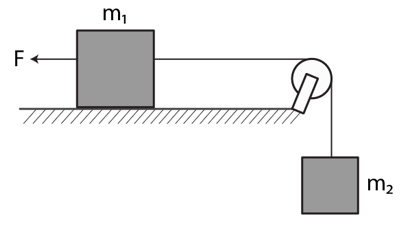Solution: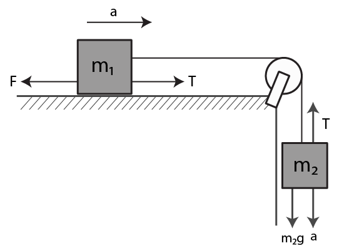Force = F = m2 (g/2) ……(given)

From free body diagram:

T – F = m1 a [for mass m_1]

=> T – m2g/2 = m1a …(1)

m2g – T = m2a ….(2) [for mass m_2]

Using (1) and (2)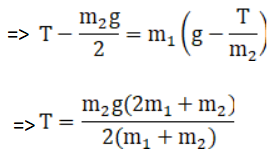We know, stress = T/A

and Young’s modulus = Y = stress/strain

Therefore, strain = T/AY

= [m2g(2m1+m2)]/[2AY(m1+m2)]

Question 10: A sphere of mass 20 kg is suspended by a metal wire of u stretched length 4 m and diameter 1 mm. When in equilibrium, there is a clear gap of 2 mm between the sphere and the floor. The sphere is gently pushed abide so that the wire makes an angle θ with the vertical and is released. Find the maximum value of θ so that the sphere does not rub the floor. Young modulus of the metal of the wire is 2.0 × 1011 N m-2. Make appropriate approximations.

Solution:

At the point of release, the tension = T = mg + mv2/r

Force which brings elongation is centrifugal force = F = mv2/r …(1)

From work energy theorem,

(1/2)mv2 = mgr(1 – cosθ)

or v2 = 2gr(1-cosθ)

(1)=> F = (m/r) 2gr(1-cosθ) = 2mg(1-cosθ)

Again, Young’s modulus = Y = FL/AI

=> Y = [2mg(1-cosθ)L]/AI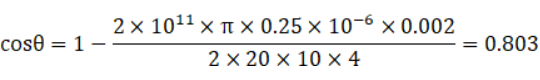=> θ = cos-1 (0.803) or 36.40 (approx)

Question 11: A steel wire of original length 1 m and cross-sectional area 4.00 mm2 is clomped at the two ends so that it lies horizontally and without tension. If n load of 2.16 kg is upending from (he middle point of the wire, what would be its vertical depression?

Y of the steel = 2.0 × 1011 N m-2. Take g = 10 ms-2.

Solution: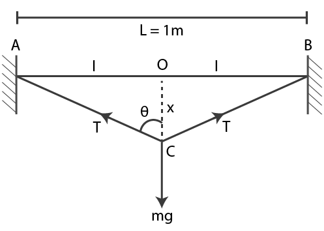From the figure,

cos θ = x/√[x2+I2]

= (x/I)(1 – x2/2I2 + …..)

[Using binomial theorem]

If x<< I, x2/I2 = 0

=> cos θ = (x/I)

Again, change in length,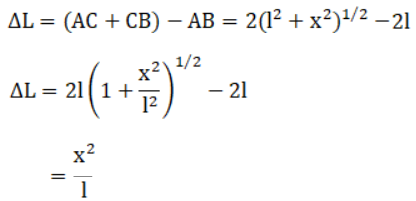Now, Using Young’s modulus,

T = (YA ΔI)/L

=> T = 8 x 105 x (x2/I)

Forces are balance, 2T cos θ = mg

=> 2 x 8 x 105 x (x2/I) x (x/I) = 2.16 x 10

=> x = 1.5 cm

Question 12: A topper wire of cross-sectional area 0.01 cm is under tension of 20 N. Find the decrease in the cross-sectional area Young modulus of copper = 1.1 × 1011 N m-2 and Poisson ratio = 0.32.

[Hint: ΔA/A = 2(Δr/r)]

Solution:

The longitudinal strain= T/AY

Where T = tension, A = cross-sectional area and Y = Young’s modulus

=> longitudinal strain = 20/[0.01×10-4x1.1×1011] = 1.82 x 10-4

The area of circular section be of the diameter D, A = πD2/4

=> dA = 2πD dD/4

=> dA = 2 x 0.01 x 5.82 x 10-5

= 1.164 x 10-6 cm2

Question 13: Find the increase in pressure required to decrease the volume of a water sample by 0.01%. Bulk modulus of water = 2.1 × 109 N m-2.

Solution:

Let v be initial volume and v’ be the final volume.

Change in volume = ΔV = -(v – v’)

and pressure, P = -B(Δv/v)

= B(1 – v’/v)

Therefore, dP = B (dv’/v)

We are given that, dv’/v’ = (0.01/100)

=> dP = B x dv’/v’ x v’/v

[Assuming, v’/v = 1]

=> dP = B (dv’/v’)

= 2.1 x 109 x (0.01/100

= 2.1 x 105 N/m2

Question 14: Estimate the change in the density of water in ocean at a depth of 400 m below the surface. The density of water at the surface 1030 kg m-3 and the bulk modulus of water = 2 × 109 N m-2.

Solution:

The bulk strain at depth h:

Δv/v = P/B = ρgh/B

Now, the density at depth is ρ’ = ρ (v/v’)

V’ is compressed volume at depth h and v’ = v – Δv

=> ρ’ = ρ x [v/(v-Δv)]

= ρB/(B-ρgh)

= [1030x2x109]/[2×109-(1030x10x400)]

= 1032 kg/m3

Therefore, change in density = 1032 – 1030 = 2 kg/m3

Question 15: A steel plate of face area 4 cm2 and thickness 0.5 cm is fixed rigidly at the lower surface. A tangential force of 10 N is applied on the upper surface. Find the lateral displacement of the upper surface with respect to the lower surface. Rigidity modulus of steel = 8.4 × 1010 N m-2.

Solution:

Shearing strain = Δx/d = Δx/0.0005 = 200 Δx

Shearing Stress = F/A = 10/(4×10-4) = 25000 N/m2

Rigidity modulus = (shearing stress)/(shearing strain)

=> 8.4 x 1010 = 25000/200Δx

=> Δx = 1.5 x 10-9 m

Question 16: A 5.0 cm long straight piece of thread is kept on the surface of water. Find the force with which the surface on one side of the thread pulls it Surface tension of water = 0.076 N m-1.

Solution:

Length of thread = L = 5 cm or 0.05 m

Surface tension of water = S = 0.076 N/m

Therefore, force due to surface tension: F = SL = 0.076 x 0.05

= 3.8 x 10-3 N

Question 17: Find the excess pressure inside (a) a drop of mercury of radius 2 mm (b) a snap bubble of radius 4 mm and (c) an air bubble of radius 4 mm formed inside a tank of water. Surface tension of mercury, soap solution and water are 0.465 N m-1, 0.03 Nm-1 and 0.076 N m-1 respectively.

Solution:

(a) excess pressure inside a drop of mercury of radius 2 mm:

P = 2S/r = [2×0.465]/0.002 = 465 N/m2

(b) excess pressure inside a snap bubble of radius 4 mm

P = 4S/r = [4×0.03]/0.004 = 30 N/m2

(c) excess pressure inside an air bubble of radius 4 mm formed inside a tank of water.

P = 2S/r = [2×0.076]/0.004 = 38 N/m2

Question 18: Consider a small surface area of 1 mm2 at the top of a mercury drop of radius 4.0 mm. Find the force exerted on this area (a) by the air above it (b) by the mercury below it and (c) by the mercury surface in contact with it. Atmospheric pressure = 1.0 × 105 Pa and surface tension of mercury = 0.465 N m-1. Neglect the effect of gravity. Assume all numbers to be exact.

Solution:

(a) Force exerted by air above:

F = PA = 105 x 10-6 = 0.1 N

(b) Force exerted by the mercury below the surface area:

Let P’ be the required pressure,

=> P’ = Po + 2T/r

Now, F = P’A = (Po + 2T/r)r

= (0.1 + (2×0.465)/(4×10-3)) x 10-6

= 0.10023 N

(c) Force exerted by the mercury surface in contact with it:

F = PA = (2T/r) A

= (2×0.465)/(4×10-3)

= 0.00023 N

Question 19: The capillaries shown in figure (below) have inner radii 0.5 mm, 1.0 mm and 1.5 mm respectively. The liquid in the beaker is water. Find the heights of water level in the capillaries. The surface tension of water is 7.5 × 10-2 N m-1.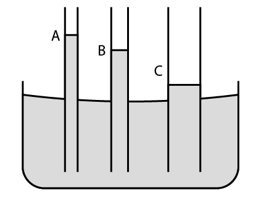Solution:

Surface tension of water = T = 7.5 x 10-2 N/m

Considering cos θ = 1

Radius of capillary A = rA = 0.5 mm = 0.5 x 10-3 m

Height of water level in capillary A:

hA = [2T cosθ]/[rA ρg]

= [2×7.5×10-2]/[0.5×10-3x1000x10]

= 3 x 10-2 m or 3 cm

Radius of capillary B = rB= 1 mm = 1 x 10-3 m

Height of water level in capillary B:

hB = [2T cosθ]/[rB ρg]

= [2×7.5×10-2]/[1×10-3x1000x10]

= 15 x 10-3 m or 1.5 cm

Radius of capillary C = rC = 1.5 mm = 1.5 x 10-3 m

Height of water level in capillary C:

hC = [2T cosθ]/[rC ρg]

= [2×7.5×10-2][1.5×10-3x1000x10]

= 15/1.5 x 10-3 m or 1 cm

Question 20: The lower end of a capillary tube is immersed in mercury. The level of mercury in the tube is found to be 2 cm below the outer level. If the same tube is immersed in water, up to what height will the water rise in the capillary?

Solution:

We know, The rise or depression in capillary = h= [2T cosθ]/[rρg]

Let the angle of mercury, θ = 0o

depression in mercury = -2 cm = -0.02 cm (Given)

For mercury = hhg = -0.02 = [2 Thg]/[rρhg g] …(1)

Let hw be the rise in water.

hw = [2Tw]/[rww g] ….(2)

Dividing (2) by (1), we get

hw/hhg = (0.075/0.465) x 13.6

= 2.19

Now, height of the water level = hw = 2 x 2.19 = 4.38 cm

Question 21: A barometer is constructed with its tube having radius 1.0 mm Assume that the surface of mercury in the tube is spherical in shape. if the atmospheric pressure is equal to 76 cm of mercury, what will be the height raised in the barometer tube? The contact angle of mercury with glass = 135° and surface tension of mercury = 0.465 N m-1. Density of mercury 13600 kg m-3.

Solution:

Rise of mercury column = h = [2 T cosθ]/[rρg]

Substituting given values,

h = [2×0.466xcos130o]/[0.001x13600x10]

= -0.005 m or -0.5 cm

[The negative sign is used as mercury goes down in a capillary tube.]

Height of mercury in the tube = h+H = -0.5 + 76 = 75.5 cm

Question 22: A capillary tube of radius 0.50 mm is dipped vertically in a pot of water. Find the difference between the pressure of the water in the tube 5.0 cm below the surface and the atmospheric pressure. Surface tension of water = 0.075 N m-2.

Solution:

Pressure = p = 2T/r

Where T = surface tension = 0.075 N/m (given)

and r = Radius of the capillary tube = 0.5 mm or 0.0005 m (given)

=> p = 300 N/m2

Pressure at a depth of 5 cm = p’ = ρgh = 1000 x 9 x 0.05 490 N/m2

Let P be the pressure of water just below surface, then

Difference in pressure : ΔP = (P + p’) – (P + p)

= (P + 490) – (P + 300)

= 190 N/m2

Question 23: Find the surface energy of water kept in a cylindrical vessel of radius 6.0 cm. Surface tension of water 0.075 J m-2.

Solution:

Area of surface = A = π r2

where r = 6 cm = 0.06 m (given)

=> A = 0.0113 m2

Now, surface energy = E = SA = 0.075 x 0.0113 = 8.4 x 104 J

Question 24: A drop of mercury of radius 2 mm is split into 8 identical droplets. Find the increase in surface energy. Surface tension of mercury 0.465 J m-2.

Solution:

Volume of drop = v = (4/3)πr3

Volume of each drop after division = v’ = v/8 = (1/6)πr3

Now the new radius of each drop be r’:

(4/3)πr’3 = (1/6)πr3

or r’ = r/2

The total area of all droplets= A’ = 8 x 4πr2‘ = 8πr2

Therefore, increase in area = 8πr2 – 4πr2 = 4πr2

Therefore, increase in energy = E = S x (increase in area) = 2.34 x 10-5 J

[S = 0.465 J m-2 and r = 0.002 cm]

Question 25: A capillary tube of radius 1 min is kept vertical with the lower end in water. (a) Find the height of water raised in the capillary. (b) If the length of the capillary tube is half the answer of part (a), find the angle θ made by the water surface in the capillary with the wall.

Solution:

Let h be the height of water raised in the capillary.

h = [2T cosθ]/rρg ….(1)

Here T = Surface tension of water = 0.076 N/m, θ = 0^o, r = 0.001,ρ = 1000 and g = 10]

=> h = 15 cm

(b) length of capillary, h’ = h/2 …(A), then

h’ = [2T cosθ]/rρg …(2)

Dividing (2) by (1)

h’/h = cos θ

using (A)

or θ = 60 degrees

Question 26: The lower end of a capillary tube of radius 1 mm is dipped vertically into mercury. (a) Find the depression of mercury column in the capillary. (b) If the length dipped inside is half the answer of part (a), find the angle made by the mercury surface at the end of the capillary with the vertical. Surface tension of mercury = 0.465 N m-1 and the contact angle of mercury with glass = 135o.

Solution:

(a) depression of mercury column in the capillary

h = [2T cosθ]/rρg

= [2×0.465xcos135o]/[0.001x13600x9.8]

= 4.9 cm

(b) Let φ be the angle made by mercury.

new height is h’ = h/2

Now, h’ = [2T cosφ]/rρg

From above equations, h’/h = cosφ/cos(135 o)

=> cosφ = -0.3535

or φ = 111 degrees (approx)

Question 27: Two large glass plates are placed vertically and parallel to each other inside a tank of water with separation between the plates equal to 1 mm. Find the rise of water in the space between the plates. Surface tension of water = 0.075 N m-1.

Solution:

The weight of water in unit volume = W = ρ h dg x 1

and given force = F = 2TI = 2 x 0.0075 x 1 = 0.150 N

Now, W = ρ h dg = 1000h x 0.001 x 10 = 10h N

or 10h = 0,150

or h = 0.15/10 = 1.5 cm

Question 28: Consider an ice cube of edge 1.0 cm kept in a gravity-free hail. Find the surface area of the water when the ice melts Neglect the difference in densities of ice and water.

Solution:

Volume of ice cube of edge = V = 1 cm3 = 10-6 m3

keeping in mind that, volumes do not change when state changes.

As gravity has no effect here, the water after melting will form a sphere,

(4/3)πr3 = 10-6

or r = [[10-6 x3]/4π]1/3 m

Now, The surface area of the sphere

A = 4πr2

Using value of r, and solving we get

A = (36π)1/3 cm2

Question 29: A wire forming a loop is dipped into soap solution and taken out so that a film of soap solution is formed. A loop of 6.28 cm long thread is gently put on the film and the film is pricked with a needle inside the loop. The thread loop takes the shape of a circle. Find the tension in the thread. Surface tension of soap solution = 0.030 N m-1.

Solution:

The loop will tale circular shape after pricking. Radius can be calculated by using relation,

l = 2πR

=> R = (6.28)/[2×3.14]

= 1 cm or 10-2 m

2T sin(dθ) force inward direction is balanced by surface tension force in outward direction.

Therefore, 2T sin(dθ) = length of arc x surface tension

for small angle, sin(dθ) = dθ

2T dθ = 2R dθ x S

Where S is the surface tension.

Now, T = SR

= 0.030 x 10-2

= 3 x 10-4 N

Question 30: A metal sphere of radius 1 mm and mass 50 mg falls vertically in glycerin. Find (a) the viscous force exerted by the glycerin on the sphere when the speed of the sphere is 1 cm s‑1, (b) the hydrostatic force exerted by the glycerin on the sphere and (c) the terminal velocity with which the sphere will move down without acceleration. Density of glycerin = 1260 kg m-3 and its co-efficient of viscosity at room temperature = 8.0 poise.

Solution:

(a) viscous force = F = 6πηrv

= 6×0.8×3.14×0.001×0.01

= 1.5 x 10-4 N

(b) The hydrostatic force exerted by the glycerin on the sphere:

Force = FB = (4/3) πr3 x ρg

= (4/3) x 3.14 x 0.0013 x 1260 x 9.8

= 5.2 x 10-5 N (approx)

(c) Terminal velocity with which the sphere will move down without acceleration

viscous force= F = 6πηrv = 6π x 0.8 x 0.001 x v = 0.0048 πv

The upwards force is due to the buoyant force in glycerin.

FB = 5.2 x 10-5 N

and the weight downwards, Fm = 50 x 10-6 x 9.8

= 4.9 x 10-4 N

As per situation, there should be no acceleration downwards:

F + FB = Fm

=> 0.0048πv + 5.2 x 10-5 = 4.9 x 10-4

=> v = 0.029 m/s

=> v = 2.9 cm/s

Question 31: Estimate the speed of vertically falling raindrops from the following data. Radius of the drops 0.02 cm, viscosity of air = 1.8 × 10-4 poise, g = 9.9 × 10 m s-2 and density of water = 1000 kg m-3.

Solution:

Viscous force is balanced by the weight:

6πηrv = ρ vg

=> v= [1000 x (4/3) πr3 x 9.9]/[6πηr]

=> v = [2000×0.0022x1.1]/[1.8×10-5] = 4.9 m/s

Question 32: Water flows at a speed of 6 cm s-1 through a tube of radius 1 cm. Coefficient of viscosity of water at room temperature is 0.01 poise. Calculate the Reynolds number. It a steady flow?

Solution:

Reynolds number = N = ρv(2r)/η

= [1000×0.06x2x0.01]/[0.001]

N = 1200 (approx)